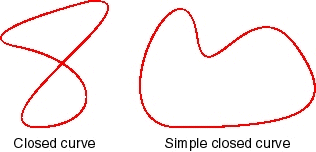# Intuition

Geometry Level 2You can create a closed curve of length $l$ by drawing any path of length $l$ that connects to its start. Some examples are given above.

Let the maximum area for a closed curve of length 1 be $A$. Find $\lfloor1000A\rfloor$.

Clarification: the symbol $\lfloor x \rfloor$ denotes the largest integer less than or equal to $x$.

×

Problem Loading...

Note Loading...

Set Loading...